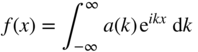# 2Derivation of the Uncertainty Principle

Consider a sinusoidal wave along the x ‐axis having amplitude a and wavelength λ . Its corresponding wave number k is defined as. We can express the wave asIf an electron is described by this wave it will have infinite spatial extent. A spatially localised wave packet may be obtained mathematically by adding a series of component sinusoidal waves together, each sinusoidal wave having a unique wavelength. This forms a Fourier series.

Consider the following sum of wavesThis may be written as an integral in the case of a continuum of wavelengths:

A2.1Assume, for simplicity, that only a range of wavelengths of the component waves exists such thatand outside of this range a(k) = 0.

Now we obtainThe amplitude of this wave is now in the form of a sinc function. We will approximate the width of the sinc function ...

Get Principles of Solar Cells, LEDs and Related Devices, 2nd Edition now with the O’Reilly learning platform.

O’Reilly members experience live online training, plus books, videos, and digital content from nearly 200 publishers.